Post

# Genetic Algorithm

In computer science and operations research, a genetic algorithm (GA) is a metaheuristic inspired by the process of natural selection that belongs to the larger class of evolutionary algorithms (EA). Genetic algorithms are commonly used to generate high-quality solutions to optimization and search problems by relying on biologically inspired operators such as mutation, crossover and selection.

## I. Notion of Natural Selection

The process of natural selection starts with the selection of fittest individuals from a population. They produce offspring which inherit the characteristics of the parents and will be added to the next generation. If parents have better fitness, their offspring will be better than parents and have a better chance at surviving. This process keeps on iterating and at the end, a generation with the fittest individuals will be found.

This notion can be applied for a search problem. We consider a set of solutions for a problem and select the set of best ones out of them.

Five phases are considered in a genetic algorithm:

• Initial population
• Fitness function
• Selection
• Crossover
• Mutation

## II. Environment: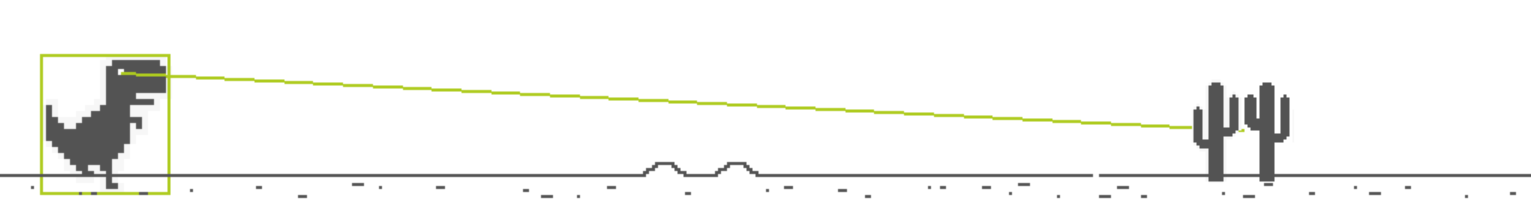This environment is based on the “Chrome Dinosaur Game,” which I rebuilt using Pygame and Numpy (More on this repo)

The agent of the game is a Dino, who knows 3 actions: run, jump or duck to dodge many kind of obstacles to stay alive as long as possible. Initially, Dino is set up with the state ”run” and he will be ready for actions depends on these types of obstacles. This Environment

Our idea is to use a neural network whose output is a matrix O size 3×1. The coefficients of this matrix correspond to 3 actions: jump , duck and run respectively. Dino will decide which action to perform depending on the highest value of the corresponding index. The input of this neural network is a vector state S size (5 × 1) correspond to 5 parameters

1 2 3 4 5 dino y: the y coordinate of Dino object x: the x coordinate of the current obstacle object y: the y coordinate of the current obstacle distance to ob: the distance from Dino to current obstacle game speed: The moving speed of the obstacles 

Action will be base on the ouput of the neural network. Given the state S in current frame, we will have:

$O = W2 × ReLU(W × S) \text{ with } ReLU(W × S) = max(0, W × S)$

## II. Genetic Algorithm

### 1. Initial population

The initial population is generated by randomly choosing coefficients for weight matrix W and W2 for each individual. The size of the population (the number of individuals) and the number of generations can be modified.

1 2 self.W = np.random.randn(16,5) self.W2 = np.random.randn(3,16) 

### 2. Fitness function

To evaluate fitness of individuals in population, we follow these 2 criteria:

• Survival time: We know that obstacles will appear and move toward Dino continuously. It means that the longer the survival time of each individual, the more obstacles that individual will pass and then the higher fitness the individual is.
• The appropriateness of action: Our target is to help Dino perform the most suitable action in all situation. For example, Dino just jumps or ducks when it meets ”challenging” obstacles (Low, Mid, Giant Bird or any types of cactus). Hence, an individual which always jumps or ducks will have the lower fitness than the one whose actions are appropriate. Furthermore, jumping or ducking continuously can not help Dino to have high score if it encounters some obstacles such that only one correct action is required to pass (we have mentioned above)

In conclusion, if we denote F is the fitness (score) of an individual, then for each frame:

$F = \begin{cases} F + 1, & \text{if the default action "Run" is active} \\ F + 0.01, & \text{if the action "Jump" or "Duck" is active multiple times} \end{cases}$

### 3. Selection

Top individuals of the previous generation with highest fitness are chose to produce offspring (through crossover and mutation) for the next generation.

1 2 3 4 5 6 7 8 9 def evaluate(self): fitness = [self.fitness(dino) for dino in self.gen] self.gen_best = np.array(self.gen)[np.argsort(fitness)][-5:] self.best_fitness = np.array(fitness)[np.argsort(fitness)][-5:] if self.best_fitness[-1] > self.best_score: self.best_score = self.best_fitness[-1] self.reset() self.w = self.gen_best[-1].W self.w2 = self.gen_best[-1].W2 

### 4. Crossover

Uniform crossover is chose to produce new offspring. We chose this method to maintain the diversity of the children and prevent premature convergence. The crossover between two good solutions may not always yield a better or as good a solution. Since parents are good, the probability of the child being good is high. If offspring is not good (poor solution), it will be removed in the next iteration during “Selection” process.

1 2 3 4 5 6 7 def crossover(self,dino1,dino2): child = Dinosaur() choice1 = np.random.randint(2, size = dino1.W.shape).astype(bool) choice2 = np.random.randint(2, size = dino1.W2.shape).astype(bool) child.W = np.where(choice1,dino1.W,dino2.W) child.W2 = np.where(choice2,dino1.W2,dino2.W2) return child 

### 5. Mutation

We modified some random coefficient in offspring’s weight matrices W and W2 after crossover to ensure the diversity of the population and also prevent premature convergence.

1 2 3 4 5 6 7 8 9 def mutation(self,dino,mutation_rate=0.05): dummy = Dinosaur() choice1 = np.random.choice([1.,0.],p = [1 - mutation_rate, mutation_rate],\ size = dino.W.shape).astype(bool) choice2 = np.random.choice([1.,0.],p = [1 - mutation_rate, mutation_rate],\ size = dino.W2.shape).astype(bool) dino.W = np.where(choice1,dino.W,dummy.W) dino.W2 = np.where(choice2,dino.W2,dummy.W2) return dino 

## III. Evaluation

I train it for 100 generations, each generations have 100 individuals.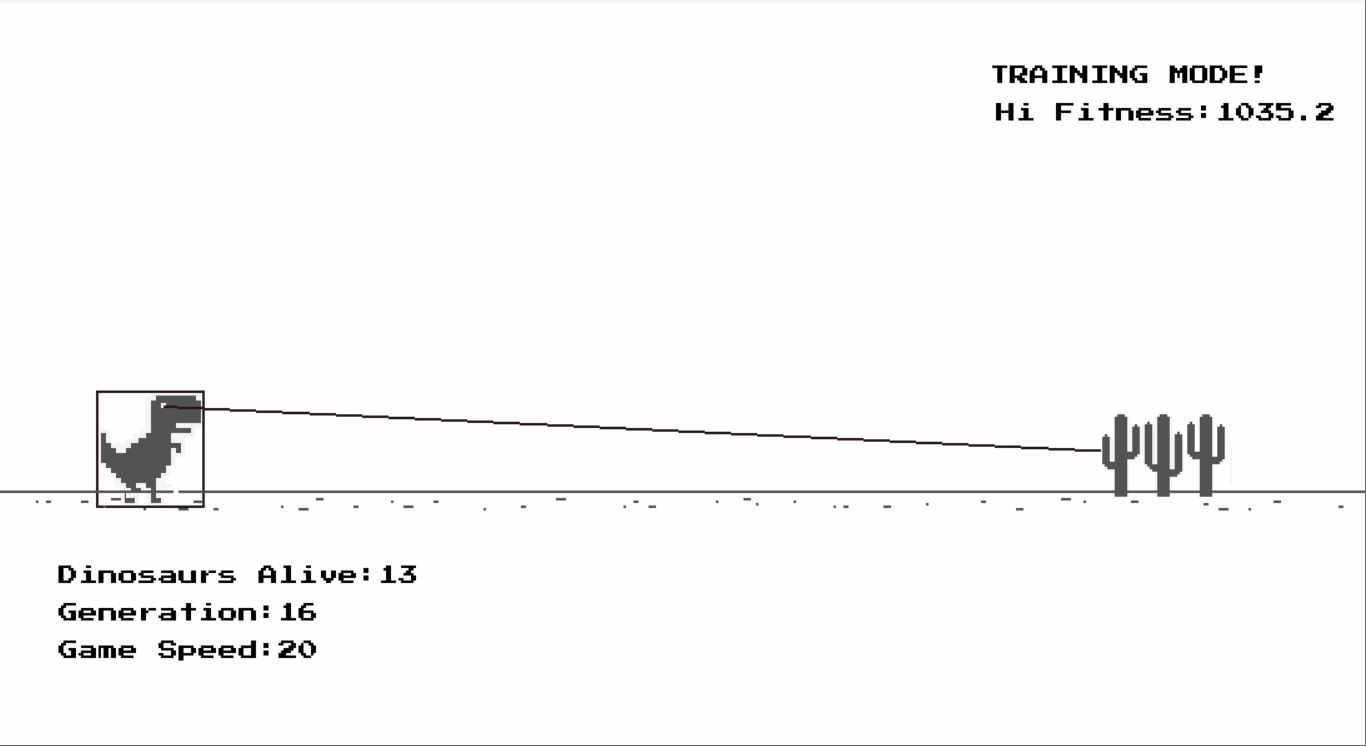### 1. Normal Mode

The line graph below illustrates the average score (fitness) of each generation after running the Training Mode 10 times: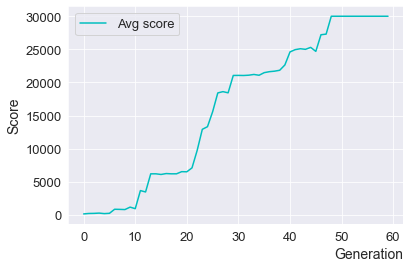In all 10 times we ran the Training Mode, the algorithm was convergent (score > 50000 or run infinitely) and had a very good result in Evaluate Mode.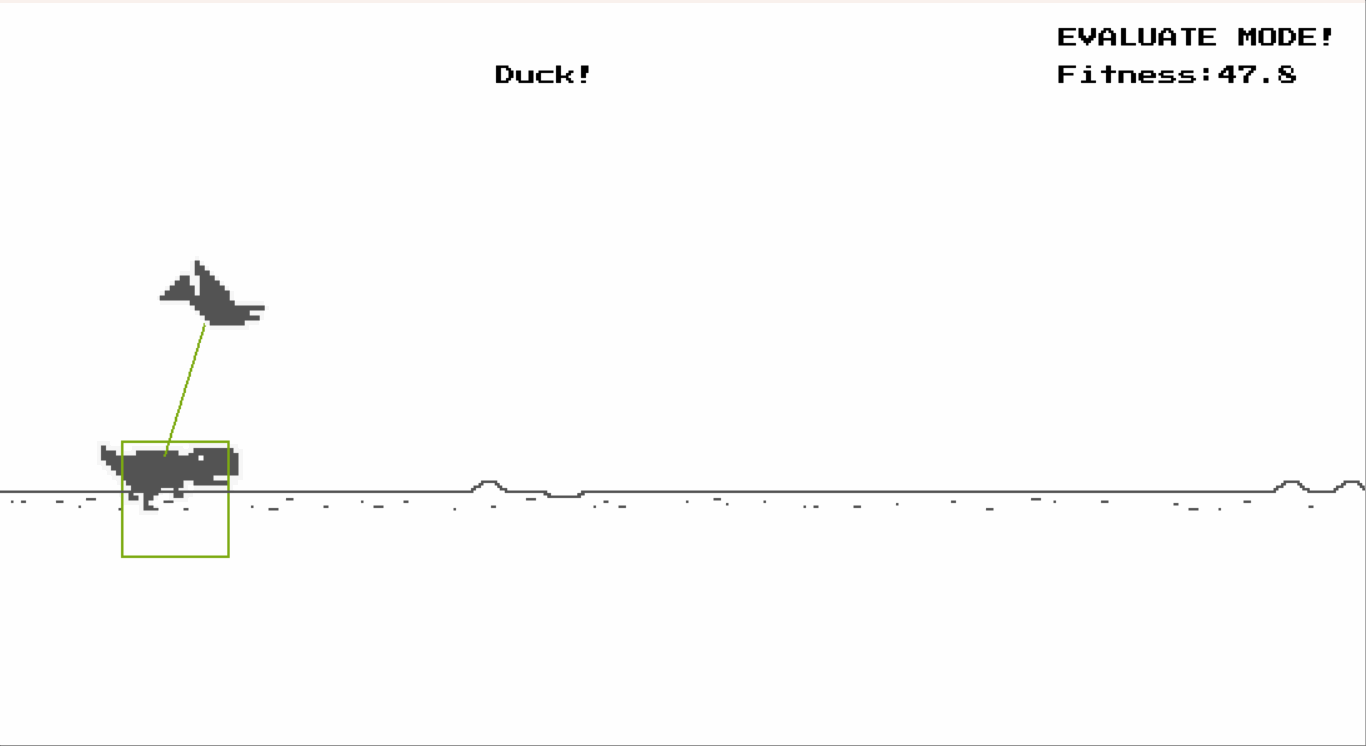### 2. Human vs GA

After training, we have evaluated the performance by controlling an agent side by side with another agent controlled by Genetic Algorithm. The result is very positive since this algorithm can easily surpass human in late game, when the difficulty and speed is increasing and too hard for human to control.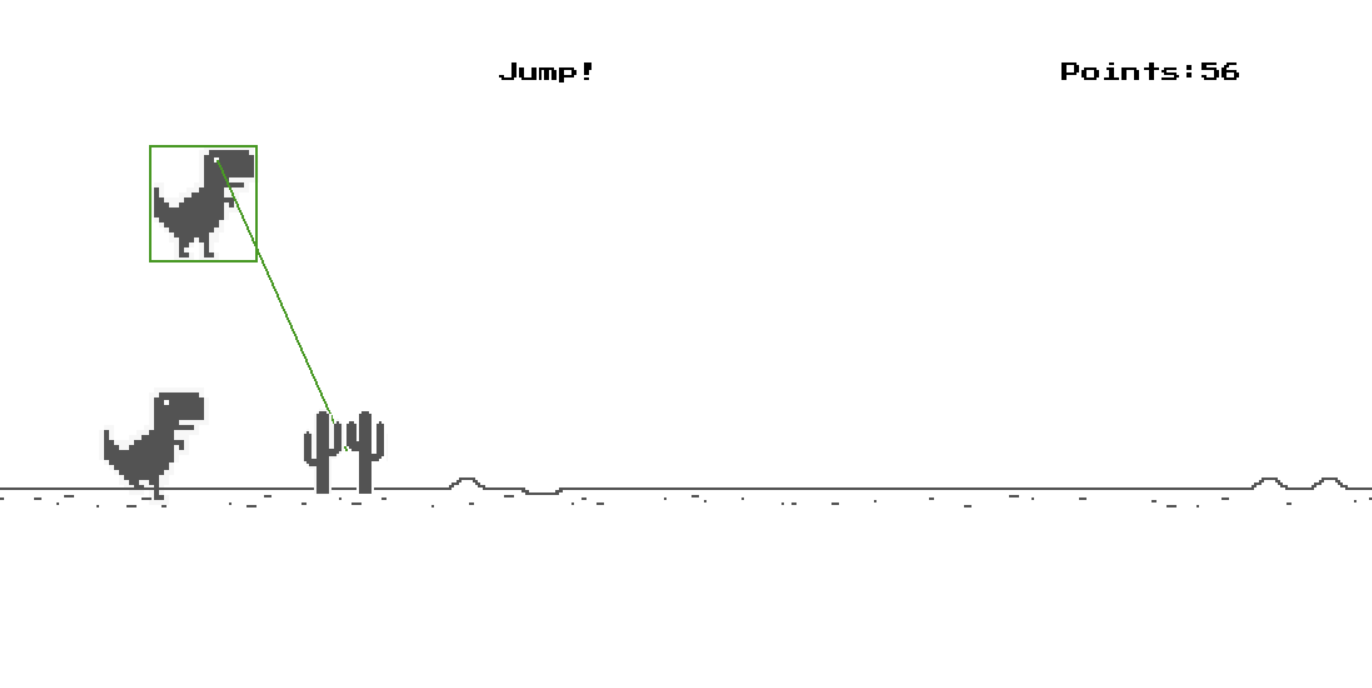### 3. GA vs If-else bot

Following our individual’s fitness evaluation section, the higher the score is, the more efficiency in decision-making the algorithm is. This graph demonstrates the comparing result between 2 algorithms: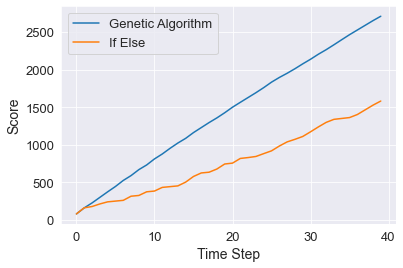## IV. Conclusion

In summary, the study of genetic algorithms provides us with a fascinating insight into the field of evolutionary computation. By simulating the processes of natural selection and genetic variation, we can explore the power of iterative optimization techniques. Genetic algorithms offer a practical and intuitive approach to problem-solving, allowing us to tackle complex, real-world challenges.